Question

The half-life of 131I is 8.04 days. (a) Convert the half-life to seconds. s (b) Calculate the decay constant for this isotope. s−1 (c) Convert 0.650 μCi to the SI unit the becquerel. Bq (d) Find the number of 131I nuclei necessary to produce a sample with an activity of 0.650 μCi. 131I nuclei (e) Suppose the activity of a certain 131I sample is 7.10 mCi at a given time. Find the number of half-lives the sample goes through in 40.2 d and the activity at the end of that period. (Enter your answer for the number of half-lives to at least one decimal place.) half-lives mCi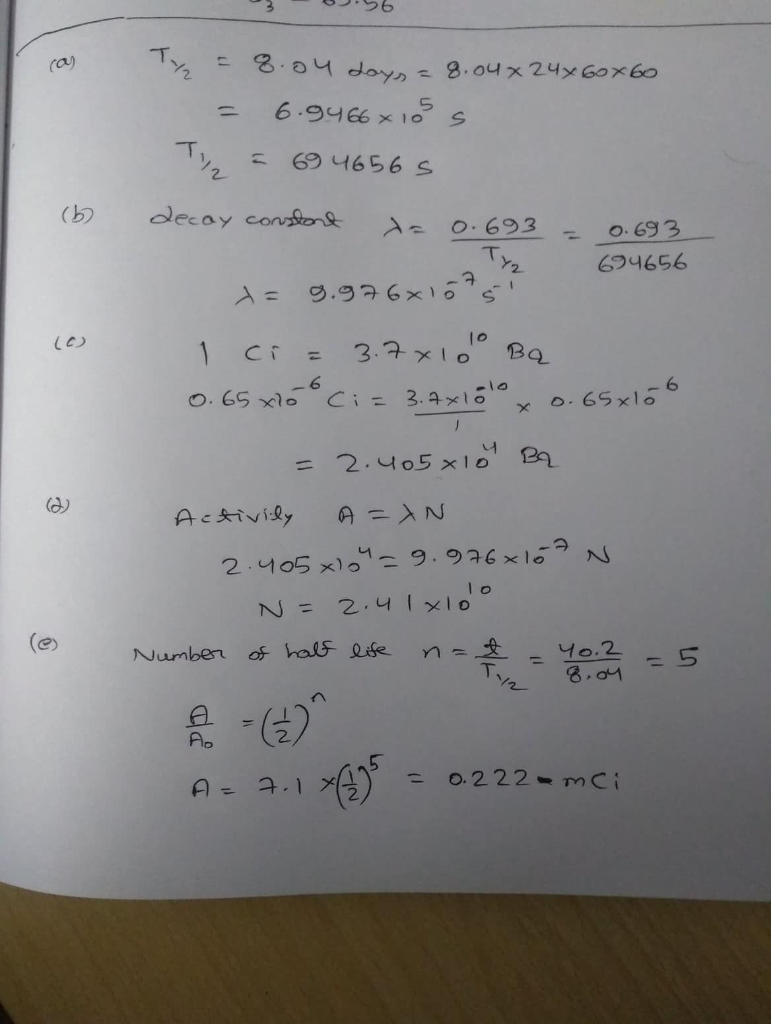#### Earn Coins

Coins can be redeemed for fabulous gifts.

Similar Homework Help Questions
• ### The half-life of 52Mn is 5.59 days. (a) Convert the half-life to units of seconds. (b)...

The half-life of 52Mn is 5.59 days. (a) Convert the half-life to units of seconds. (b) What is the decay constant (in s−1) for this isotope? (c) Suppose a sample of 52Mn has an activity of 0.510 µCi. What is this activity expressed in the SI unit of becquerels (Bq)? (d) How many 52Mn nuclei are needed in the sample in part (c) to have the activity of 0.510 µCi? E. Now suppose that a new sample of 52Mn has...

• ### The half-life of 131I is 8.04 days. On a certain day, the activity of an iodine-131...

The half-life of 131I is 8.04 days. On a certain day, the activity of an iodine-131 sample is 6.36 mCi. What is its activity 37.8 days later?

• ### 1. Calculate the activity of a 200.0 g sample of natural potassium. Express your answer both...

1. Calculate the activity of a 200.0 g sample of natural potassium. Express your answer both in Curies (Ci) and in Becquerel (Bq). 1 Bq = 1 nuclei s−1 1 Ci = 3.700×1010 nuclei s−1 2. Technetium-99m is a metastable form of technetium-99 (isotopic mass = 98.9062547 u) and has a half-life of 6.0058 hours. How many grams of Tc-99m are required to have an activity of 50.0 μCi? 3. Ytterbium-169 is a radioactive isotope. 10.0 μg of pure Yb-169...

• ### 1. The half-life of an isotope of phosphorus is 14.2 days. If a sample contains 3.96e16...

1. The half-life of an isotope of phosphorus is 14.2 days. If a sample contains 3.96e16 such nuclei, determine its activity.? 2. A 234-mCi sample of a radioactive isotope is purchased by a medical supply house. If the sample has a half-life of 17.4 days, how long will it keep before its activity is reduced to 28.9 mCi?

• ### Radioactive Decay - Half-life and Activity 1 Radioactive decay - Half-life Time 0 1000 21 31...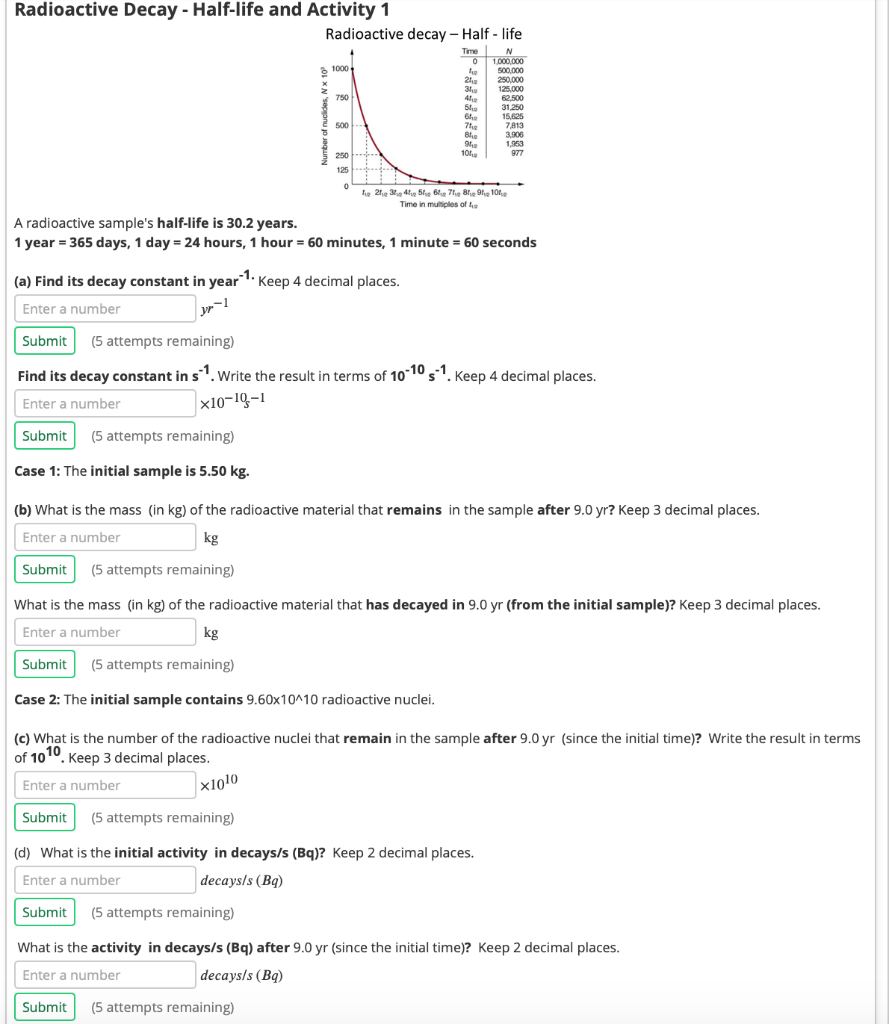Radioactive Decay - Half-life and Activity 1 Radioactive decay - Half-life Time 0 1000 21 31 750 N 1.000.000 500,000 250,000 125,000 62,500 31.250 15.625 7813 3.506 1.953 977 51 6 500 7 BI . 101 250 125 0 tie 21.234.41516171819, 1012 Time in multiples of A radioactive sample's half-life is 30.2 years. 1 year = 365 days, 1 day = 24 hours, 1 hour - 60 minutes, 1 minute = 60 seconds (a) Find its decay constant in year...

• ### 8. Fermium-253 has a half-life of 0.334 seconds. A radioactive sample is considered to be completely...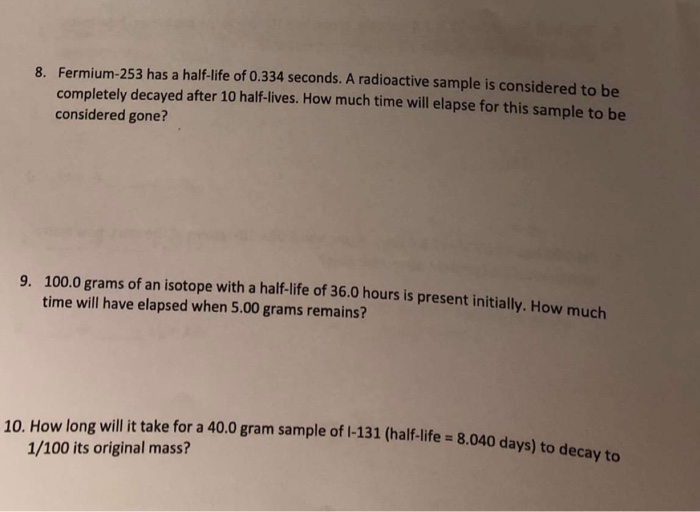8. Fermium-253 has a half-life of 0.334 seconds. A radioactive sample is considered to be completely decayed after 10 half-lives. How much time will elapse for this sample to be considered gone? 9. 100.0 grams of an isotope with a half-life of 36.0 hours is present initially. How ma time will have elapsed when 5.00 grams remains? 131 (half-life = 8.040 days) to decay to 10. How long will it take for a 40.0 gram sample of 1-131 (half-life =...

• ### Calculate rate constant and half-life for the radium decay.

The activity of a radioactive sample is the number of nuclear disintegrations per second, which is equal to the first-order rate constant times the number of radio-active nuclei present. The fundamental unit of radioactivity is the curie (Ci), where 1 Ci corresponds to exactly 3.70 x 10^10 disintegrations per second. This decay rate is equivalent to that of 1 g of radium-226. Calculate the rate constant and half-life for the radium decay. Starting with 1.0g of the radium sample, what...

• ### Phas half life of 1.23 x 10 second. [Ans: 1.41x1017nuclei, 7.9x 1010 decay/s, 1.2x1016nuclei] If we...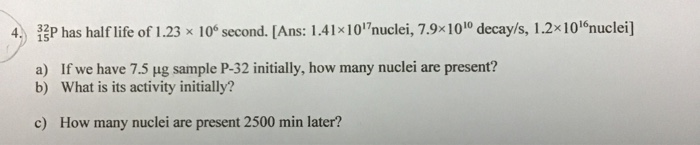Phas half life of 1.23 x 10 second. [Ans: 1.41x1017nuclei, 7.9x 1010 decay/s, 1.2x1016nuclei] If we have 7.5 ug sample P-32 initially, how many nuclei are present? a) What is its activity initially? b) How many nuclei are present 2500 min later? c)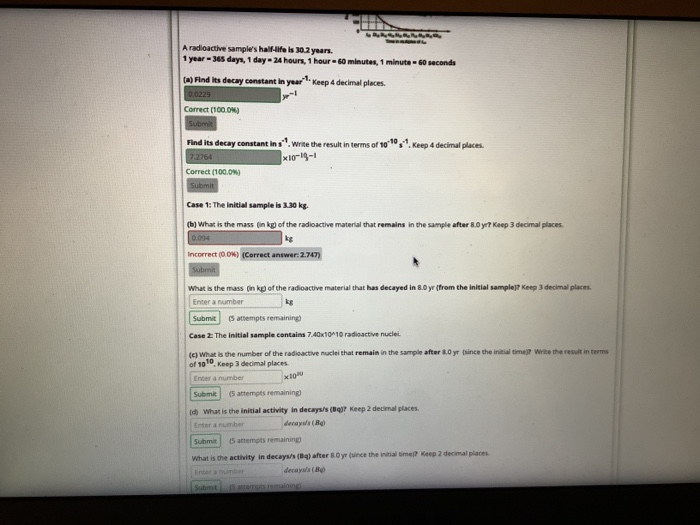please help with b-d! *10-19-1 A radioactive sample's half-life is 30.2 years 1 year - 365 days, 1 day - 24 hours, 1 hour 60 minutes, 1 minute - 60 seconds (a) Find its decay constant in year 1. Keep 4 decimal places. 0.6229 Correct (100,0%) Submit Find its decay constant in sl. Write the result in terms of 10 10,9 Keep 4 decimal places. 7.2764 Correct (100.0 Submit Case 1: The Initial sample is 3.30 kg. (b) What is...

• ### 11 please Seconds Minutes Hours Days Years 10. List 3 nuclides with a half-life of less than 1 minute, and 3 with a half-life of more than 10 years. 12 Nels 11. Do this exercise on a separate shee...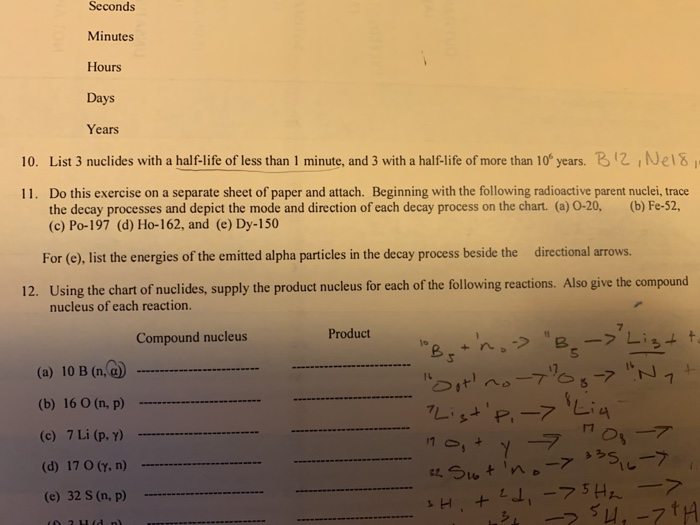11 please Seconds Minutes Hours Days Years 10. List 3 nuclides with a half-life of less than 1 minute, and 3 with a half-life of more than 10 years. 12 Nels 11. Do this exercise on a separate sheet of paper and attach. Beginning with the following radioactive parent nuclei, trace (b) Fe-52, the decay processes and depict the mode and direction of each decay process on the chart. (a) 0-20, (c) Po-197 (d) Ho-162, and (e) Dy-150 rs For...